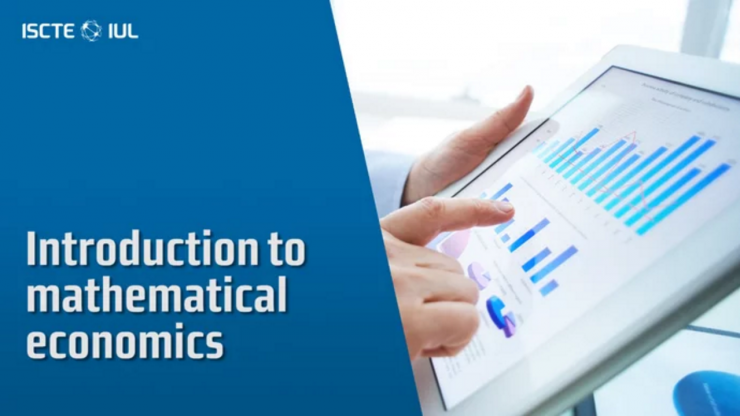Introduction to Mathematical Economics
3 Módulos | 10 Minutos | 1 ECTSIntroduction to Mathematical Economics

Welcome to Mathematical Economics! You should start each module by watching the introductory video, then going through the material in the pdf slides. At the end of each module there is a short quiz with simple questions.

The course is meant as a revision and slides are, therefore, summaries of the main results. A more thorough presentation of the material can be found in Chiang and Wainwright, 2005, Fundamental Methods of Mathematical Economics (chapters are indicated in the slides). You should study the in-chapter examples and do the end-of-section exercises in the book to assess your problem-solving skills and prepare for the work you will have to do during the program.